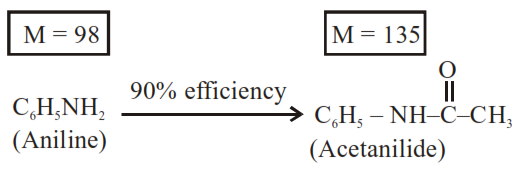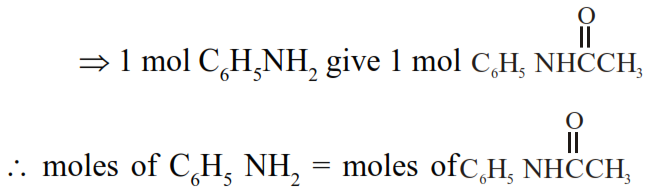# 1.86 g of aniline completely reacts to form acetanilide.`
Question:

$1.86 \mathrm{~g}$ of aniline completely reacts to form acetanilide. $10 \%$ of the product is lost during purification. Amount of acetanilide obtained

after purification (in $\mathrm{g}$ ) is__________ $\times 10^{-2}$

Solution:Given $1.86 \mathrm{~g}$$\Rightarrow \frac{1.86}{93}=\frac{W_{\text {ace tan ilide }}}{135}$

$\Rightarrow \mathrm{W}_{\text {acclanilide }}=\frac{1.86 \times 135}{93} \mathrm{~g}=2.70 \mathrm{~g}$

But efficiency of reaction is $90 \%$ only

$\therefore$ Mass of acetanilide produced $=2.70 \times \frac{90}{100} \mathrm{~g}$

$=2.43 \mathrm{~g}$

$=243 \times 10^{-2} \mathrm{~g}$

$\Rightarrow x=243$